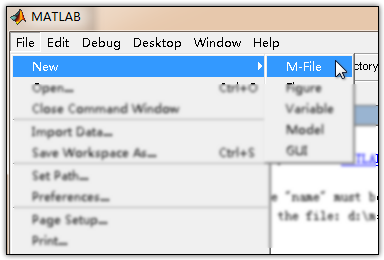• Matlab函数定义和调用 Matlab 自定义函数的五种方式 命令文件/函数文件+ 函数文件 多个 M 文件 自定义函数的五种方式 函数文件 + 子函数 一个 M 文件 inline 无需 M 文件 符号表达式 + subs 方式 无需 M 文件 字符串...
• for i = 1:x fprintf(‘Enter the wind-speed for turbine %d’,i) ; a = input(’ : '); min = a+1 ; secmin = a+1 ; if min > a secmin = min ; min = a ; secinnum = minnum ; minnum = j ;... j =
for i = 1:x fprintf(‘Enter the wind-speed for turbine %d’,i) ; a = input(’ : '); min = a+1 ; secmin = a+1 ; if min > a secmin = min ; min = a ; secinnum = minnum ; minnum = j ; j = j+1 ;
elseif secmin > a
secmin = a ;
secinnum = j ;
j = j+1 ;
end
ws(i) = a ;
sum = sum + a ;

end
fprintf(‘The slowest wind-speed is for turbine %d with a speed of %d\n’, minnum,min) fprintf(‘The second-slowest wind-speed is for turbine %d with a speed of %d\n’,secinnum,secmin)
fprintf('The wind speeds are: ‘) for i = 1:x fprintf(’ %d ',ws(i)) end
dev = get_deviations(ws); fprintf('The deviations are: ‘) for i = 1:x fprintf(’ %d ',dev(i))
function [dev] = get_deviations(ws) x = length(ws) ; sum = 0 ; for i = 1:x sum = sum + ws(i) ; end ave = sum/x ; for i = 1:x dev(i) = ws(i) - ave ; end
展开全文• ## matlab函数定义详解

万次阅读 2014-06-28 16:23:49
但凡经过编程熏陶的人，在学习其他一门陌生... 在数学中，定义一个函数，我们需要明确定义域、值域、表达式。在matlab中，则对应为输入参数，输出参数，函数体。实际上，matlab支持多输入多输出，函数的返回值可以为一

但凡经过编程熏陶的人，在学习其他一门陌生的编程语言时，往往会与已经学过的语言相比较。而语法的比较往往是情不自禁的。在matlab中，函数的概念或许没有其他语言那么广泛，特性没有那么丰富，但基本的功能都已具备。
在数学中，定义一个函数，我们需要明确定义域、值域、表达式。在matlab中，则对应为输入参数，输出参数，函数体。实际上，matlab支持多输入多输出，函数的返回值可以为一个向量，矩阵，或者多个元素。为了让函数可以调用，需要对函数进行命名。
即包含：

输入参数
输出参数
函数名
函数体

我们以求两个数的最小公倍数，最大公约数为例，来演示matlab的函数的定义。打开matl,选择file->new->M-file在弹出的窗口中，输入以下代码


展开全文matlab
• 解决倒立摆中非线性化问题，使用s-function函数，输入为控制量为u
• ## matlab函数定义

千次阅读 2019-06-11 14:26:22
matlab定义函数，和C语言类似，定义一个从华氏度到摄氏度的函数如下 function D =F2C() for i=0: 10 F = input('==>Temperature in F:','s');%input返回的是一个字符串，需要str2num转化成数字 if isempty(F) ...
matlab定义函数，和C语言类似，定义一个从华氏度到摄氏度的函数如下
function D =F2C()

for i=0: 10
F = input('==>Temperature in F:','s');%input返回的是一个字符串，需要str2num转化成数字
if isempty(F)
break
end
F = str2num(F);
C = ((F-32).*5/9);
fprintf('Temperature in C:%f\n',C);

end
end

function D =F2C() D是返回值，F2C是函数名字，括号里是形参，这里我把函数定义为无参函数。 在 matlab中条件判断不需要括号括起来，fprintf和C语言的printf用法类似，如果%d表示小数使用科学计数法显示，用%f是用普通的数值显示。
matlab中的end代替了C语言的大括号，要注意的就是这些
展开全文• Matlab函数 函数是组织好的，可重复使用的，用来实现单一，或相关联功能的代码段。 函数能提高应用的模块性，和代码的重复利用率。你已经知道Matlab提供了许多内建函数，比如disp()。但你也可以自己创建函数，这被...
Matlab函数 函数是组织好的，可重复使用的，用来实现单一，或相关联功能的代码段。
函数能提高应用的模块性，和代码的重复利用率。你已经知道Matlab提供了许多内建函数，比如disp()。但你也可以自己创建函数，这被叫做用户自定义函数
matlab

disp(‘hello world’) hello world 总的来说，自定义函数分为两步：即定义函数和调用函数。

定义一个函数 你可以定义一个由自己想要功能的函数，以下是简单的规则：
函数代码块以 function关键词开头，后接输出变量和函数标识符名称和圆括号 ()。 任何传入参数和自变量必须放在圆括号中间，圆括号之间可以用于定义参数。 函数的第一行语句可以选择性地使用文档字符串—用于存放函数说明。 函数内容以end结束。 函数需要定义在脚本文件（*.m）中运行，不可以在命令窗口中临时创建。
语法 Matlab定义函数需要使用function关键字，一般格式如下：
matlab function 输出变量 = 函数名（输入变量） 函数体 end 这里的输入变量和输出变量都可以根据实际情况缺省。
实例 创建一个函数，用来输出“hello world!” ，步骤如下：
新建一个脚本，命名为eg1.m
matlab test(); function test() disp(‘hello world!’) end 点击顶部菜单的运行按钮，即可在命令窗口输出：
matlab

eg1 hello world! 更复杂一点的应用，我们让函数带上输入变量和输出变量：

matlab s = calc_area(4,5); function s = calc_area(w,h) s = w*h; disp([‘area=’,num2str(s)]); end 以上实例输出：
matlab

eg2 area=20 输入变量缺省 有时候，输入变量的个数不确定，但是又想将不同个数情况统一到一个函数里，即输入变量有缺省时，可以利用nargin来判断，然后给出对应的输出。例如：

matlab out(1) out(1,2) out(1,2,3) function out(a,b,c) if nargin == 1 disp(a) elseif nargin == 2 disp([a,b]) elseif nargin == 3 disp([a,b,c]) end end 上面的实例将会得到：
matlab

eg5 1

 1     2

1     2     3

可以看到，当输入不同输入变量个数时，会找到对应个数下的执行代码，返回相应的内容。
当然这种做法也存在弊端，当变量个数太多时，要写很多个判断；这种情况下可以尝试inputParser,这里我就不多解释了，有兴趣可以了解下。
函数调用 函数基本结构完成后，进行调用时，存在两种方法：
直接在函数所在脚本文件内调用（上面的例子就是这种情况）； 函数定义与调用分开，各自存为一个脚本，运行调用脚本。 法一：脚本内调用函数 直接在函数脚本内调用 直接在函数脚本内调用
如上图所示，调用函数在前，定义函数在后，点运行即可得到输出。
法二：函数脚本独立，另外写代码调用 调用函数 调用函数
定义函数 定义函数
如上图所示，函数脚本和调用函数的脚本分开了，保存在同一路径下。
在第二种方法下，也可以在命令窗口直接调用所写的函数：
命令窗口调用函数 命令窗口调用函数
这种调用与上面本质上是一样的，都是首先找到以函数名命名的脚本文件，然后再执行函数内部代码块，返回结果。在这里必须注意函数脚本名字要与函数名一致。
事实上，为了让编程变得系统化，模块化，我们不建议你采用第一种方法去写代码，因为这样不利于分析和维护代码。
而采用后面一种方法时，我们可以定义很多个函数，然后在其他脚本里调用它们，这样形成了一种模块化的关系。我们在分析代码时，注意力可以集中到主程序上，思路将更加清晰。
输出值缺省调用 在matlab的函数中，可以选择性地获取所返回的变量列表值，下面给出实例：
matlab default = out() [a,,]= out() [,b,] = out() [,,c] = out() function [a,b,c] = out() a = 1; b = 2; c = 3; end 上述实例输出结果：
matlab

eg4

default =
 1

a =
 1

b =
 2

c =
 3

可以看到，函数默认以返回的第一个变量为输出值；若想得到返回的某个值时，用变量接住，对于不需要的值，则需要用~占位，但不接收这个值，这样做的目的就是为了保证输出的序号能够匹配到。
展开全文• 以下是关于函数定义 function p = init_phi(im,type) im = dimensionz(im); %m = im(150:250,150:250); [dim1, dim2] = size(im); p = zeros(dim1+2,dim2+2); switch lower (type) case 'circle...开发语言
• matlab 初学函数定义 function [dev] = get_deviations(ws) #这个是定义函数 x = length(ws) ; sum = 0 ; for i = 1:x sum = sum + ws(i) ; end ave = sum/x ; for i = 1:x dev(i) = ws(i) - ave ; end for i = 1:x ...
• ## matlab函数定义格式

万次阅读 2012-03-30 21:24:15
总结matlab函数定义的一些内容： 1, 函数定义格式 在matlab中应该做成M文件,文件名要和你文件里的function后面的函数名一致 在File新建一个M-file 在M-file里编辑函数 格式为: function [输出实参表]=函数名...function file c
• ## matlab中函数的定义和使用

万次阅读 多人点赞 2019-05-25 22:31:00
• ## Matlab定义函数的几种方法

万次阅读 多人点赞 2017-09-07 15:20:13
1、函数文件+调用命令文件：需单独定义一个自定义函数的M文件 例：自定义求和函数 需要自定义一个 my_sum.m 的文件来存放函数 function[s]=my_sum(n) s=0; for i=1:n s=s+i; end注意：函数名（my_sum）必须...
• ## MATLAB自定义函数

万次阅读 2019-09-28 01:32:24
MATLAB自定义函数形式 function [a,b,c] = funname(x1,x2,x3) 输入变量 对于输入变量，MATLAB可以识别输入变量的个数，通过nargin来记录当前输入变量个数。 if nargin == 1 ··· elseif nargin == 2 ····...
• 详细介绍labview调用matlab函数的方法，以及过程中的注意事项。 详细介绍labview调用matlab函数的方法，以及过程中的注意事项。
• matlab支持多输入多输出，函数的返回值可以为一个向量，矩阵，或者多个元素。为了让函数可以调用，需要对函数进行命名。 function [输出变量] = 函数名称(输入变量） 即包含： 输入参数 输出参数 函数名 注释 ...
• matlab 引用定义函数时，function要放在引用底下
• MATLAB定义函数可变参数个数 使用nargin判断输入函数个数 function out=func(a,b,c,d) % nargin 表示参数个数 if nargin==4 % ... elseif nargin==3 % ... elseif nargin==2 % ... else % ... end ...
• 在做数字信号处理的时候我们会发现有很多函数没有定义，在这我整理了一个库，包含数字信号处理中所用到的函数定义库。希望能帮助到大家
• matlab的文件里面调用一个子函数，例如： test.m中内容： function a end call a; ... 在一些matlab的版本上会报错，可以用一个方法解决： test.m改成如下，然后再调用test【跟文件同名】函数。 funciton ...
• function re=add_multi(a,b,c,d) % % nargin 表示参数个数 if nargin==4 re=a+b+c+d; elseif nargin==3 re=a+b+c; elseif nargin==2 re=a+b; else error('输入有错啊！'); end ...add_multi(3,
• ## MATLAB画函数图像

万次阅读 多人点赞 2019-04-19 15:56:34
1 画图基础 （1）一元一次函数 x=0:0.1:1; y=x; plot(x,y); %图像见下图1 图1 ...
• INLINEDEF 提供了在脚本中定义函数或类的外观（减去语法突出显示），尽管包含函数或类定义的单独 M 文件实际上是自动生成的并保存在 Matlab 路径上 - 并且没有更具体的范围。 与直接创建 M 文件相比，使用 INLINEDEF...
• 最近被matlab代码生成中的数据类型折磨的死去活来，在此简单梳理一下。MATLAB的数据类型和C语言类似，都分为数值型、字符串型、数组型、结构型。主要介绍数值型数据。 1 数值型 首先数值型分为三种类型：整数、...
• MATLAB函数定义： 1、函数文件+调用函数文件：定义多个M文件： 2、函数文件+子函数：定义一个具有多个子函数的M文件 3、Inline:无需M文件，直接定义； 4.匿名函数 5、Syms+subs: 无需M文件,直接定义； 6、字符...function
• 自定义函数，tstem(hn,y)，可以用来作离散序列柄状图。
• MATLAB可以在单独的.m文件中定义函数 比如有一文件myadd.m，文件中内容为 function y = myadd(a, b) % 这里可以写函数的使用说明，前面以%开头 % 在工作区中，help myadd将显示此处的说明 y = a + b; end %可以...
• ## MATLAB函数速查手册

千次阅读 多人点赞 2018-03-25 09:06:26matlab
• 函数定义格式： function 变量名 = 函数名（n个参数） 描述语句   举例： function y = sinfun1(M) %函数功能和参数作用 x = 0 : M-1; for k = 1 : numel(x)  y(k) = sin(x(k) / (100 * pi)); end  注意：...
• MATLAB的DFT定义源程序代码 保存为M文件后，直接调用即可DFT
• varargin、varargout 定义任意个参数 nargin、nargout得到函数实际输入、输出参数个数 ...常见函数定义一般有：        无输入、无输出函数；   ......

# matlab函数定义matlab 订阅